Study Guide

# Limits

## Limits

Functions are usually written something like this: y = f(x).

x is called the independent variable, while y is called the dependent variable. The independent variable is the input. The dependent variable is the output.

Got it? Good. Let's get cracking.

• ### Functions Are Your Friend

Functions are machines. Plug the independent variable into the machine and it spits out the dependent variable.

### Sample Problem

If y = f(x) = x + 1, then as x gets larger (moves right), y gets larger also (moves up). As x gets smaller (moves left), y gets smaller also (moves down).

Here's something to play with: see what happens to y as we make x larger or smaller.### Sample Problem

If y = f(x) = 1 – x, then as x gets larger (moves right), y gets smaller (moves down). As x gets smaller (moves right), y gets larger (moves up).

### Sample Problem

Say y = f(x) = x2. We start x at -5. As x moves right, y gets smaller until x reaches 0. If, starting at 0, we keep moving x to the right, y starts getting bigger again.

With this function, in order to say whether y is increasing or decreasing as we play with x, we need to know two things: whether x is to the left or the right of zero, and whether x is being moved to the right or left.

• ### Graphing and Visualizing Limits

It's super helpful to plug numbers into the function and see the output. It's even more helpful to graph the results. Try to draw or imagine how a function actually looks. Is it really hip to be x2? Draw the graph and decide for yourself.

### Sample Question

Let y = f(x) = x3 – 2. As x gets close to zero, what does y approach?

As x approaches zero, y approaches -2. There are several different ways to say this:

• As x gets close to 0, y gets close to -2.
• As x gets close to 0, f(x) gets close to -2.
• As x approaches 0, y approaches -2.
• As x approaches 0, f(x) approaches -2.
• As x goes to 0, y goes to -2.
• As x goes to 0, f(x) goes to -2.

Each of these phrases mean the same thing. Here's yet another way to say it:

The limit of f(x) as x approaches 0 is -2.

We know what "x approaches 0" means. The limit of f(x) is the value f(x) is getting close to.

We can have x approach other numbers besides 0.

### Sample Problem

Let y = f(x) = cos(x). What is the limit of f(x) as x approaches 2π?

Moving x around, we see that as x gets closer to 2π, f(x) gets close to 1. The limit of f(x) as x approaches 2π is 1.

This is the basic idea behind limits. We look at what a function does as the independent variable, or input, gets closer and closer to some specified value.

• ### Piecewise Functions and Limits

Now we'll shake things up a bit by taking limits with piecewise-defined functions. Here's an example:What is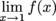?

If we draw the graph of this function, we see that it looks like the line y = x + 1 except at one point. When x = 1, instead of having y = 2 like we would expect, the point has jumped off the line up to y = 3.How does a function like this affect what we know about limits? Imagine we're taking Bruno, the ugliest dog, for a walk. We would expect him to stay on the sidewalk. We wouldn't expect him to suddenly teleport to Middle-earth, then reappear and continue on his path. He may look like Gollum, but still...

When talking about limits, we're talking about what we expect the function to be doing. We assume Bruno is approaching solid ground.In the example above, the limit is 2, because that's what we would expect the value of the function to be if we looked at values of x close to (but not equal to) 1.

We can think ofas the value that f(x) gets "close" to as x gets close to 1.

• ### One-Sided Limits

Becky has been planning her Florida vacay for months. The only thing left on her to-do list is to find a new bathing suit. She's cruising the web to find the perfect one. Eventually, she gives up on the sizing charts and hops in the car to check out what Target has to offer. She drives into the parking lot and starts looking around for a space. She finds the perfect parking spot and she decides on the best way to approach it. Assuming there aren't any parking dividers, the spot can be approached from the left (a pull through in this case) or the right. Either way, the same spot is approached. How does the parking lot at Target relate to calculus? Let's learn, fellow swim-wear seekers.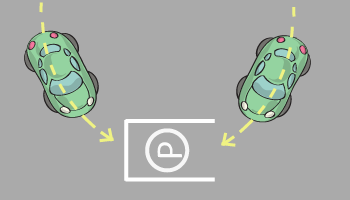Limits can be approached from the left or the right. If we're only approaching from one direction, then it's a one-sided limit.

If x is approaching 1 from the left, we write a minus sign like this: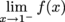to show that x is approaching 1 from the negative side. We call this a left-hand limit, since x is coming from the left.If x is approaching 1 from the right, we write a positive sign like this: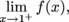to show that x is approaching 1 from the positive side. We call this a right-hand limit, since x is coming from the right.### Sample Problem

Let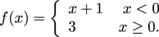Find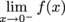and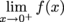.

When we graph this function, we find this:If x starts out to the left of zero, we can completely ignore the chunck of the graph at y = 3. As x moves closer to zero from the left, y gets closer to 1.

Therefore,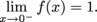.

If x starts out to the right of zero and moves closer to zero, y gets closer to 3. Then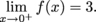.

Notice that the actual value of f(0) is irrelevant to calculating the limits. We don't care about what's actually happening at x = 0, just what's happening as we get super close to it.

When the left- and right-hand limits exist and are approaching the same value, we say the two-sided limit exists. Using symbols, if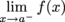=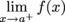= b,

then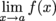= b.If the left-and right-hand limits are not approaching the same value, then we say the limit does not exist (DNE). If the two sides can't find any common ground, there really isn't any way to decide on a value for the limit. Compromise just won't work here.

• ### Limits via Tables

There's more than one way to approach (pun absolutely intended) limit problems. We've already looked at graphs and equations.

Another way to estimate the limit of a function is to use a calculator to see what the function approaches as we plug in values of x that get closer and closer to some value a. To keep things organized, now we'll use tables to get the lowdown on functions.

### Sample Problem

If f(x) = x2, estimate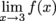.

This is how it'll go. We'll make a table. In one column we'll have values of x, and in the next we'll have the corresponding values of f(x).

First we have x approach 3 from the left.

xf(x)
2.5  6.25
2.77.29
2.97.29
2.98.41
2.998.9401
2.9998.994001

The values of f(x) in the table appear to be getting closer to 9 as x approaches 3 from the left. We'll see what happens if x approaches 3 from the right.

xf(x)
3.1 9.61
3.019.0601
3.0019.006001
3.00019.0006001

The values of f(x) appear to be approaching 9 as x approaches 3 from the right as well. We can now shout from the rooftops that, indeed,= 9.

When using tables to determine limits, there's no particular rule about what numbers to plug in for x as it approaches a number a. As long as we look at lots of values of x, and let them get really close (as in, 0.00001 close) to a, we should be fine.

• ### Limits via Algebra

Most of the time, it's more precise (and a lot faster) to find limits using algebra.

When finding a limit of the form, where f(x) is just one nice algebraic expression, the first thing to do is plug a into the function to see if it exists. If f(a) exists, then that's the answer. This won't work for piecewise functions, since there could be gaps separating the pieces of the function.

### Sample Problem

Find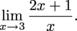.

The first thing we do is plug in 3 and see if we find a value.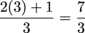That's a perfectly good fraction, therefore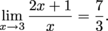Here's an example of how we might not find a solution.

### Sample Problem

Find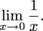.

The first thing we do is plug in 0 and see if we find a number.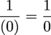= ?

The fraction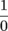is undefined, since we can't divide by zero.

### Sample Problem

Find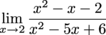.

The first thing we do is see if we can plug in 2.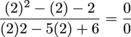When we're asked to find the limit of a quotient, if we plug in a number and find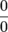there's a good chance we can do something about it: we simplify the quotient and try again.

### Sample Problem

Find.

We already tried to plug in 2 and that didn't work. We'll simplify the fraction by factoring the polynomials.

x2x – 2 = (x + 1)(x – 2)

and

x2 – 5x + 6 = (x – 2)(x – 3).

Now we can see why we gotbefore: 2 is a root of the polynomial in the numerator, and also a root of the polynomial in the denominator. Here's the point where we can do something useful: cancel the term (x – 2) from the numerator and denominator: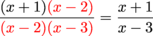.

Finally, put in 2 again: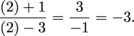We can now say= 2.

Why does this work? Let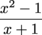Now we'll think about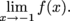.

The numerator of f(x) can be factored as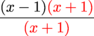which equals (x – 1) for every value of x except -1 (when x = -1, the function is undefined).

We'll say that again, because it's important. After factoring f, we see that we can think of it as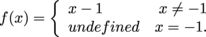.

If we graph this, we find the line x – 1 with a "hole" in the graph at x = -1 since f(-1) is undefined:

It's like the function f(x) is trying to be x – 1, but failing at one spot (poor function!). Here's the good news: since f(x) is trying to be x – 1, we can find the limit by instead finding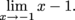.